# Catch up on the latest AI articles# Does LLM Have Causal Reasoning Capability?

3 main points
✔️ Proposed benchmark dataset to test causal inference ability of large-scale language models
✔️ 17 existing large-scale language models evaluated
✔️ Current models found to have poor causal inference ability

written by Zhijing JinJiarui LiuZhiheng LyuSpencer PoffMrinmaya SachanRada MihalceaMona DiabBernhard Schölkopf
(Submitted on 9 Jun 2023)

Subjects: Computation and Language (cs.CL); Artificial Intelligence (cs.AI); Machine Learning (cs.LG)code：The images used in this article are from the paper, the introductory slides, or were created based on them.

## Introduction

Causal reasoning ability is one of the key capabilities of human intelligence. This ability consists of two components: empirical knowledge and pure causal inference.

Large-scale language models (LLMs) can capture correlations between terms from vast amounts of linguistic data, but the ability to extract causal relationships from this data remains an important question.

In this paper, we propose a dataset CORR2CAUSE to test the ability to infer purely causal relationships.

We then investigate the current LLM's ability and whether they can improve their performance through re-learning.

## Data-set

### Sorting out terminology

Describes causal relationships based on graph theory. For example, when the relationship (edge) $e_{i,j}$ between two events (nodes) $X_i$ and $X_j$ is $X_i \rightarrow X_j$, $X_i$ is the direct cause of $X_j$. Here we assume that there is no cycle.

In other terms, when $X_i \rightarrow X_j$, we say that $X_i$ is the parent of $X_j$, and conversely $X_j$ is the child of $X_i$. When there is a directed path from $X_i$ to $X_j$, we say that $X_i$ is an ancestor of $X_j$, and conversely $X_j$ is a descendant of $X_i$.

Also, for the three elements $X_i, X_j, X_k$, when $X_k$ has a common parent $X_i, X_j$ (i.e., a common cause), $X_k$ is called a confounder; conversely, when $X_k$ is a child of $X_i, X_j$ (i.e., a common result), $X_k$ is called collider, and when $X_i \rightarrow X_k \rightarrow X_j$, $X_k$ is called a mediator.

The task is to predict from a pair of statements $\mathbf{s}$ describing correlations between $N$ elements $\mathbf{X}=\{X_1, ..., X_N\}$ and a hypothesis $\mathbf{h}$ describing causal relationships between elements $X_i$ and $X_j$ in $(\ mathbf{s}, \mathbf{h})$, the task is to predict the label $v$ that indicates whether the hypothesis is correct or not. If the hypothesis is correct, $v=1$ is predicted; if it is incorrect, $v=0$ is predicted.

### Creating Data Sets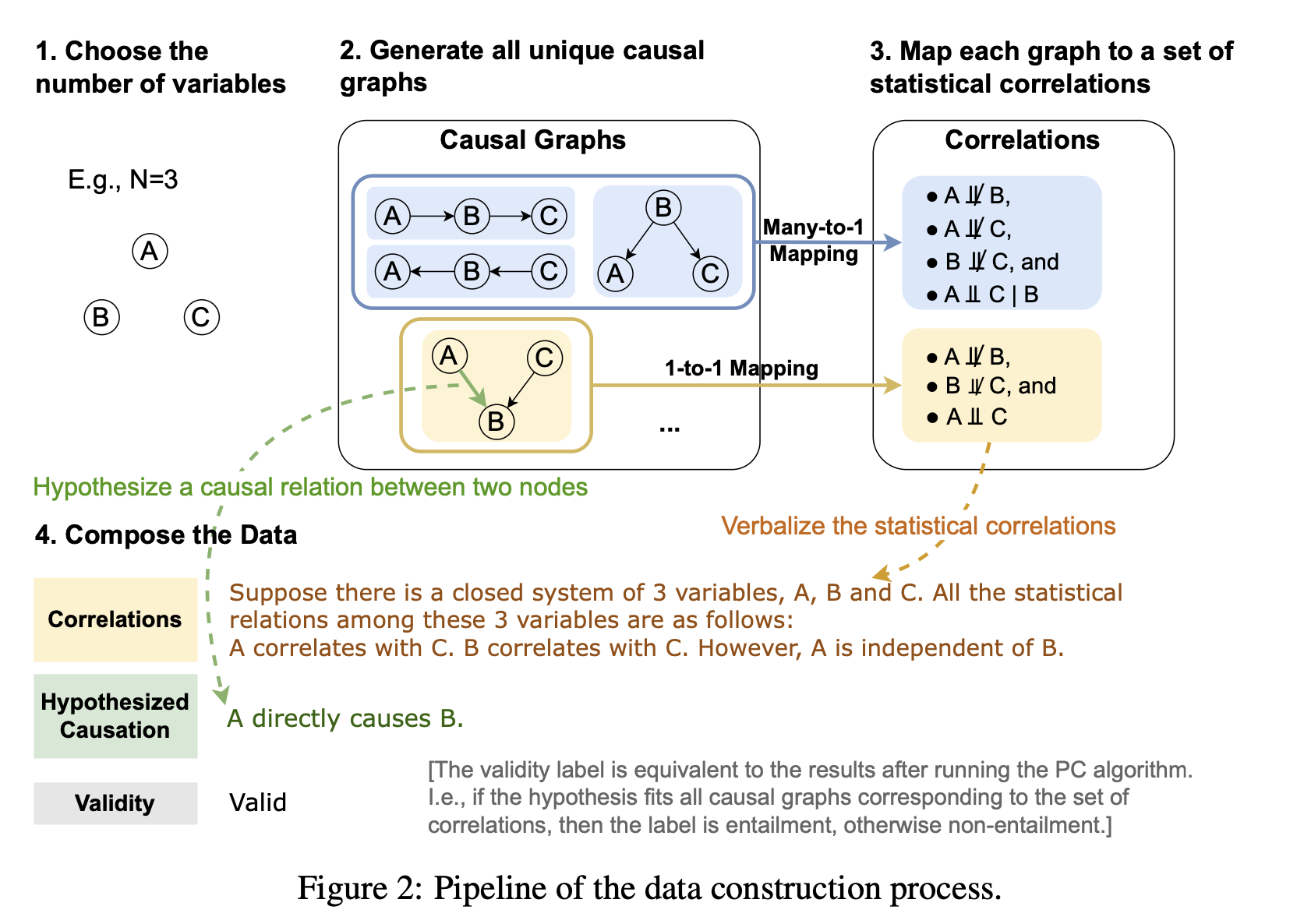The figure above is an overview of dataset creation. First, the number of elements (nodes) $N$ is determined and possible relationships (graphs) are listed. Then, two elements are extracted from each graph (relationship), and hypotheses are made about the causal relationship between them in the following six patterns.

Is-Parent: Parent or not.
Is-Child: Child or not.
Is-Ancestor: Ancestor or not. Is-Descendant:
Descendant or not. Is-Ancestor: Is an ancestor, but not a parent.
Is-Descendant: Is
a descendant, but not a chi ld. Has-Confounder: Is a confounder. Is-Confounder: Whether there is a confounder (common cause).
Has-Confounder: Whether there is a confounder (common result).
Has-Collider: Whether there is a collider (common result
).

If the hypothesis is correct, it is assigned a label of 1; if it is wrong, it is assigned a label of 0.

The relationships (graphs) and hypotheses obtained above are paraphrased in natural language as shown in the table below.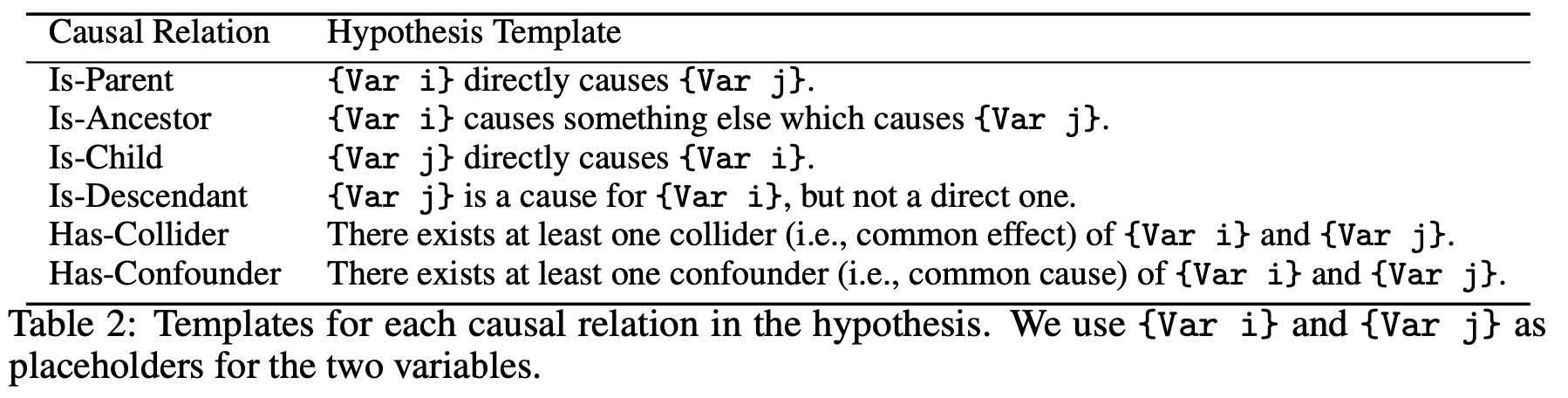### Statistical information about the dataset

The created dataset CORR2CAUSE has a total of 415944 samples, of which 411452 are used as training data. (See table below)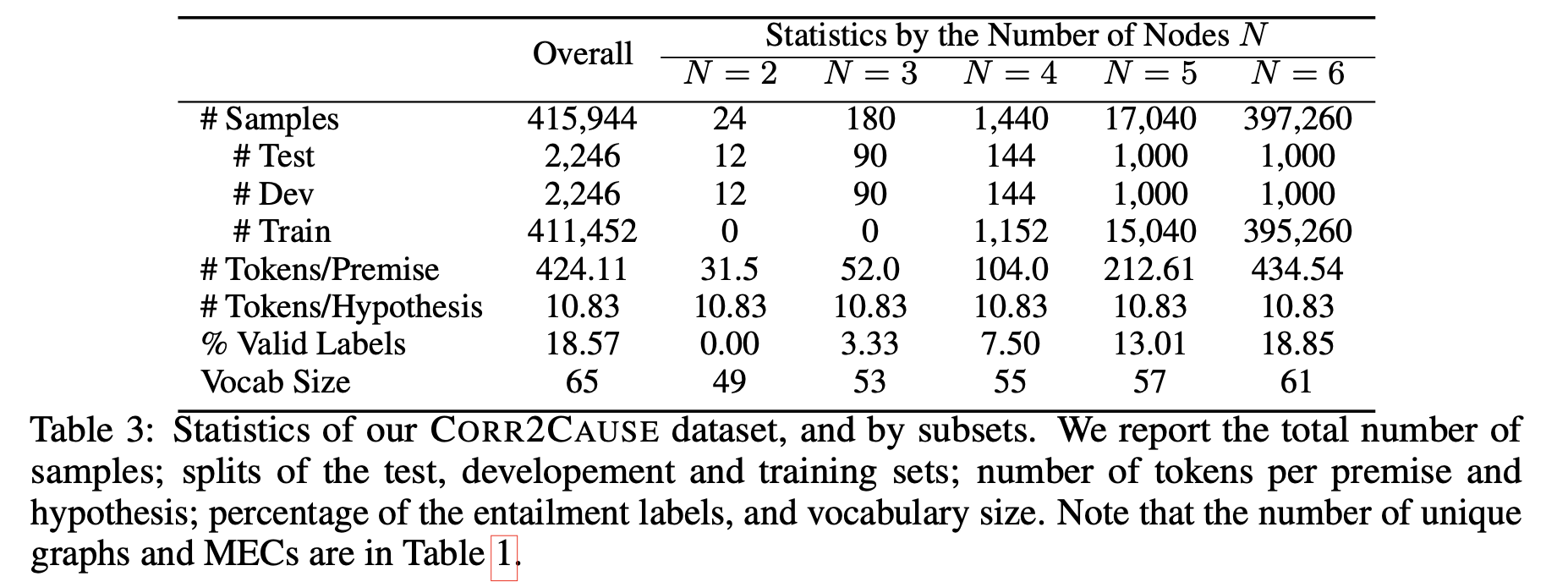## Experimental results

Several BERT-based, GPT-based, and LLaMa-based models were prepared and their performance on the CORR2CAUSE dataset was investigated.

### For trained LLMs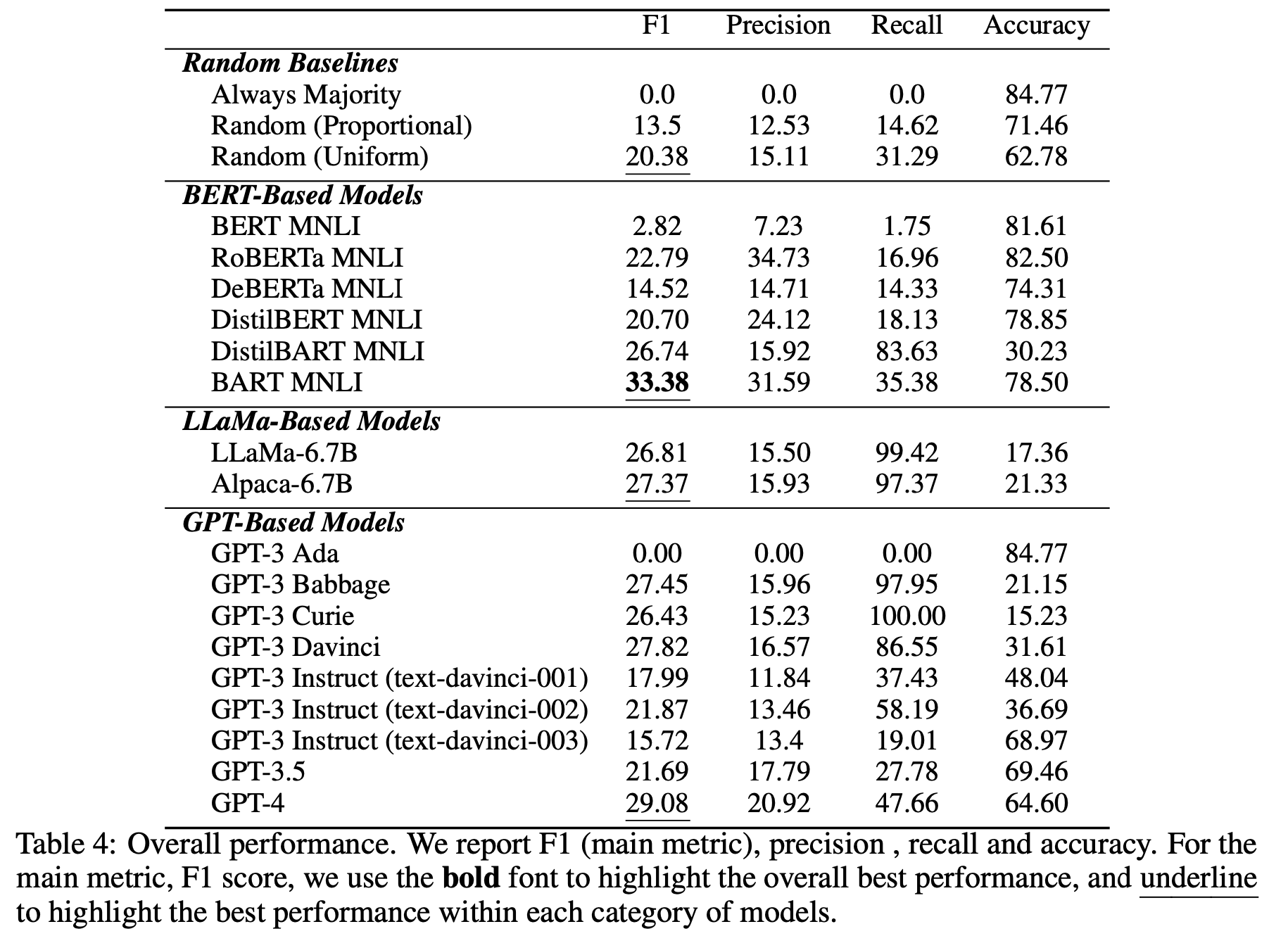The table above shows the performance of the publicly available trained LLMs. All of the models struggled with the CORR2CAUSE task, with the best one having a low 33.38% F1 score.

### If fine-tuned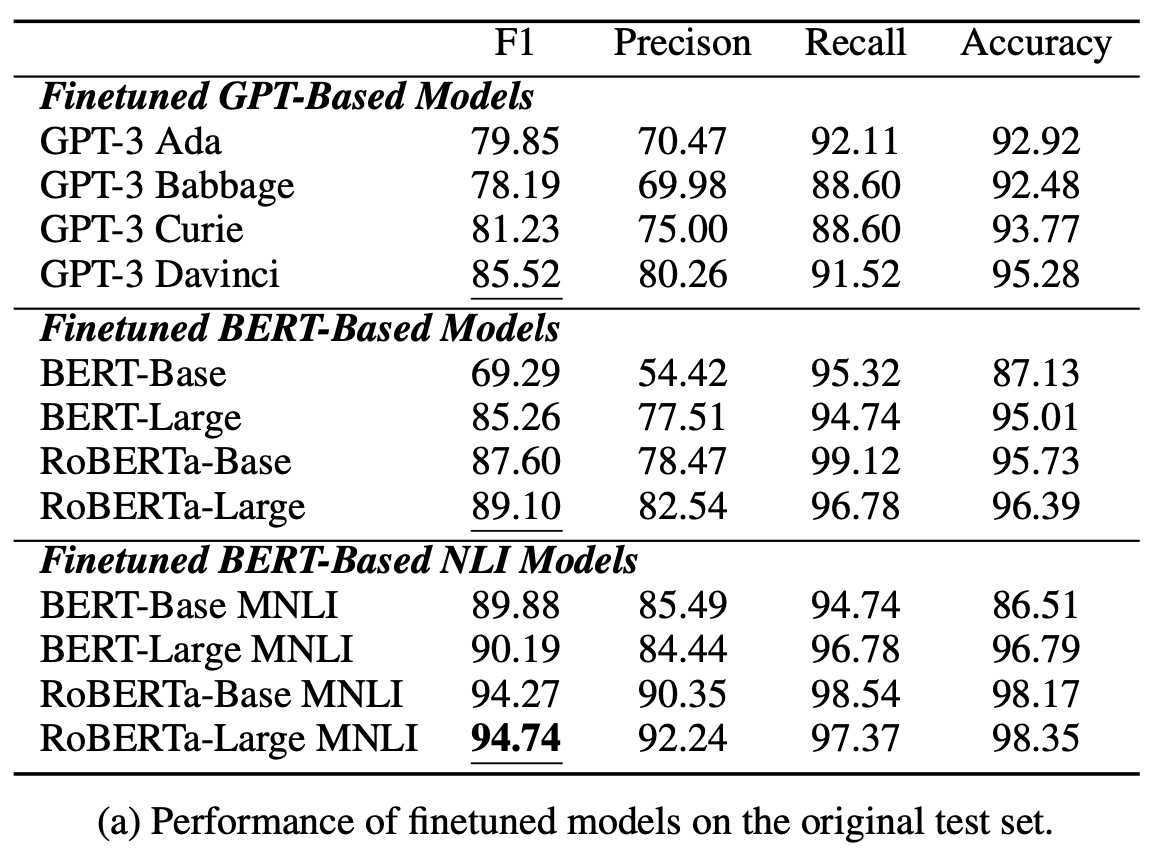The table above shows the performance of the LLM models after fine-tuning on the CORR2CAUSE dataset. At first glance, one can see that the performance of all models is significantly improved compared to before fine-tuning.

The figure below shows the performance of RoBERTa-Large MNLI, which performed the best, for each causal pattern, showing that it was strong for the Is-Parent, Is-Descendant, and Has-Cofounder patterns, but struggled a bit with the Has-Collider Is-Parent, Is-Descendant, and Has-Cofounder patterns, while it struggles slightly against Has-Collider.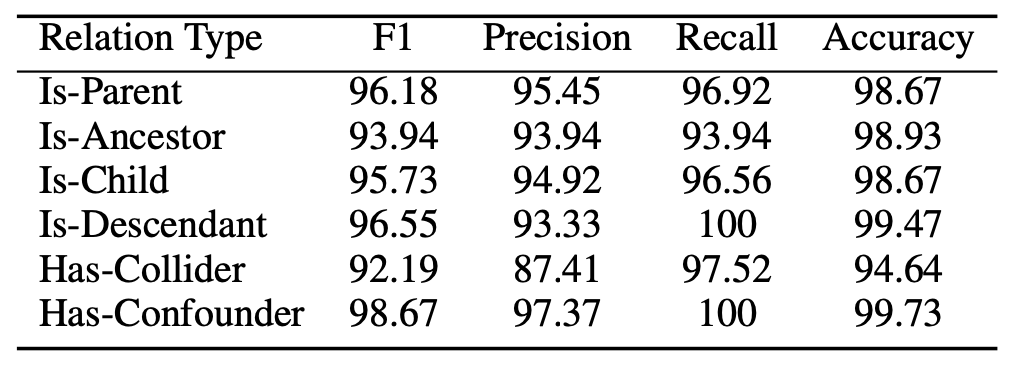### Robustness Study

Because fine-tuning greatly improved performance, the following question arose: Can the model robustly infer causality? To investigate this question, two additional experiments were conducted.

Paraphrasing: Rephrase the hypothesis and ask the model again, as shown in the figure below.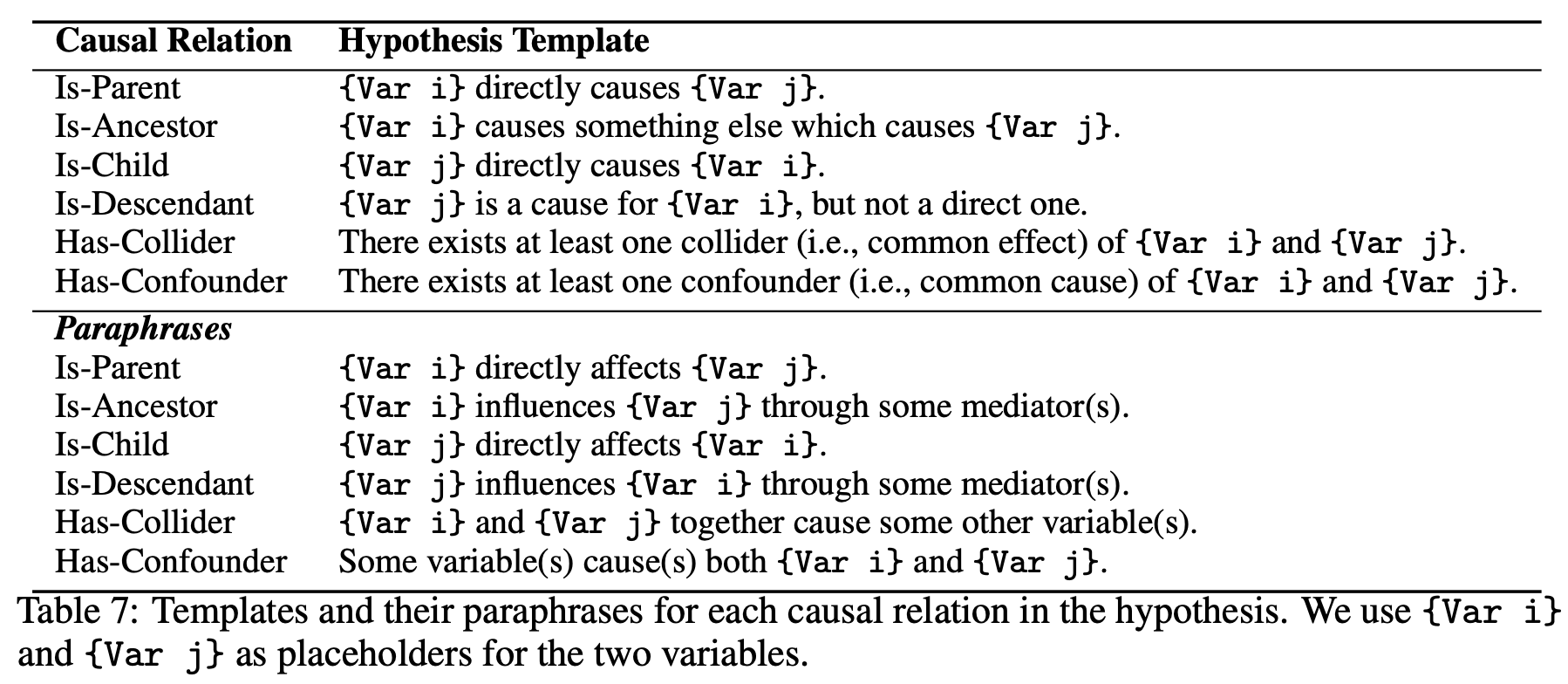Variable substitution: Simply replace the letters of the variable names used from A, B, C to X, Y, Z.

The results of the additional experiments are shown on the right side (b) of the following table. We can see that paraphrasing and variable substitution significantly reduce the performance of all models. We can see that the models do not generalize to data outside the distribution of the training data.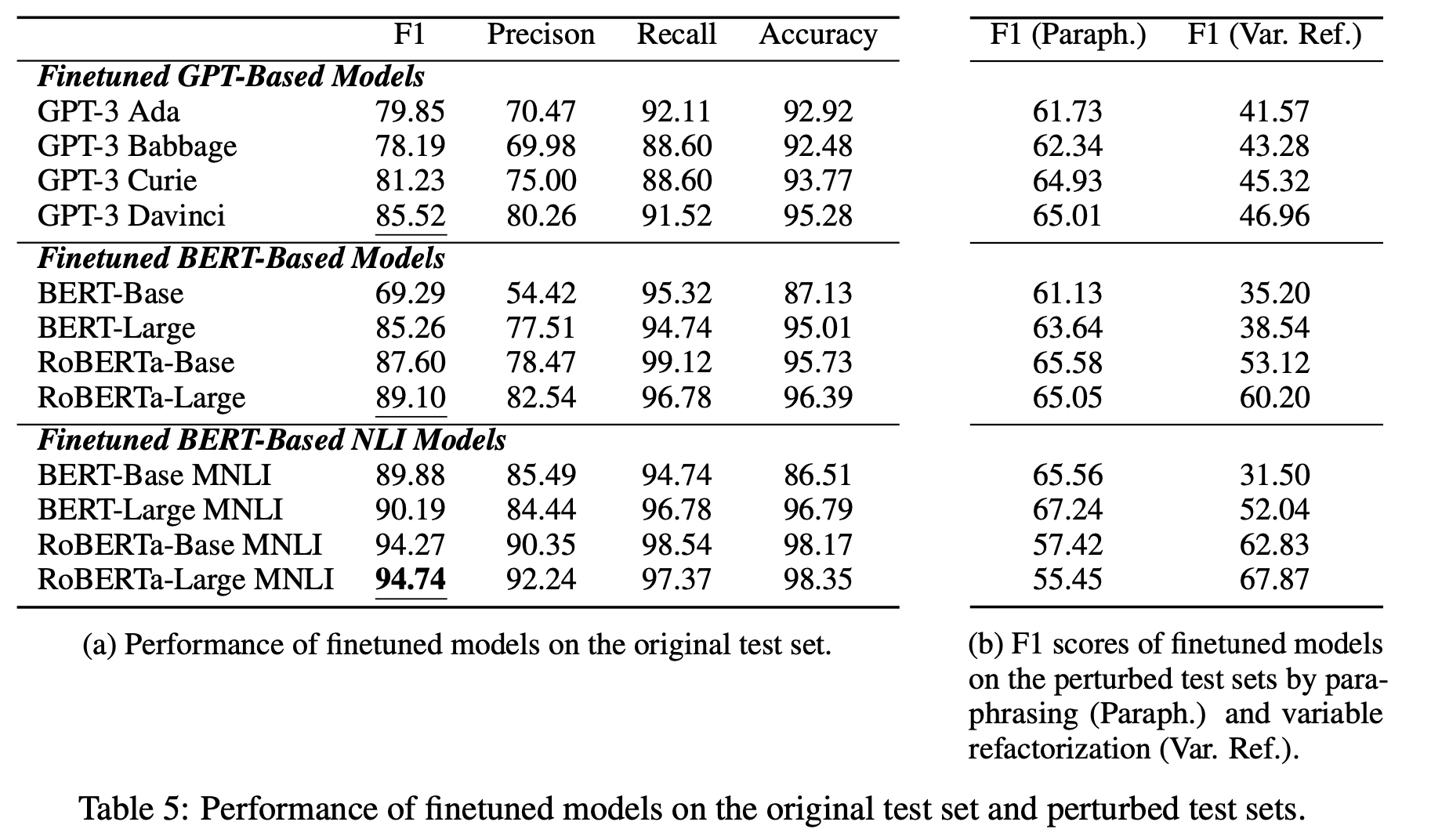## Discussion

In this paper, we proposed a dataset CORR2CAUSE to investigate causal inference ability. This dataset is a large dataset consisting of over 400,000 samples. Since it was not used to train the original LLM, it is an appropriate dataset for investigating causal inference capability. Limitations include the limited number of nodes (2 to 6) and the fact that hidden common causes are not assumed.

Experiments on this dataset show that existing LLMs struggle with causal inference; while fine-tuning improves performance, a slight change in wording can cause performance to drop, a phenomenon that will require more detailed investigation in future research.

## Summary

In this issue, we introduced a paper that proposed a dataset CORR2CAUSE for investigating the causal inference capability of LLMs. It seems that the current LLMs still have poor causal inference performance. How can we improve the causal inference capability of LLMs? Continued research is needed.

If you have any suggestions for improvement of the content of the article,